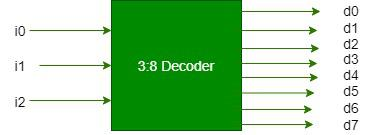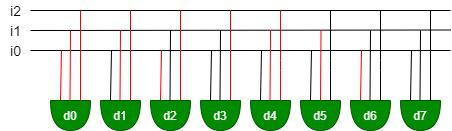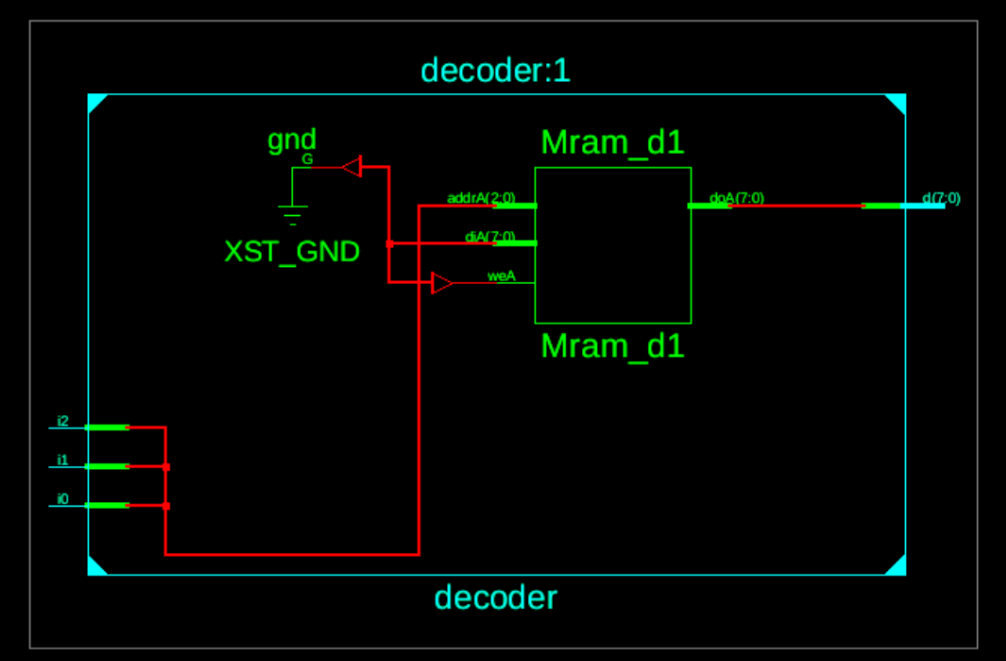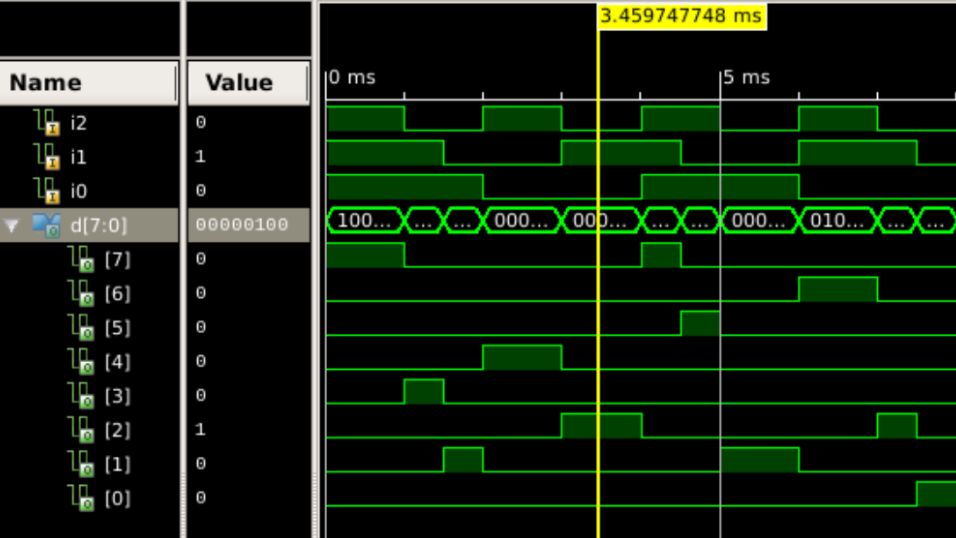Open in App
Not now

# Implementation of Decoder Using VHDL

• Difficulty Level : Expert
• Last Updated : 27 Oct, 2022

The decoder is a combinational circuit consists of ‘n’ no of input lines and ‘2^n’ no of output lines. It decodes the original signal from encoded input signal. Here, a structure of 3:8 line decoder is implemented using hardware level programming language VHDL( VHSIC Hardware Description Language).

This VHDL programming language is used to design models of digital system by Dataflow, Behavioral and Structural style of modeling. VHDL gives us the features like we can generate the circuit diagram as our requirements and can generate wavefronts from which we can check the systems input-output values and compare with it’s original truth-table. Moreover, we can perform various Sequential & Concurrent activities within the model as VHDL gives a wide range of descriptive capabilities within a model. VHDL consists of total five types of design units–> Entity , architecture, configuration, package and package body.The logical circuit diagram is given below:On the above circuit diagram red line are representing a connection with negation(connected with a nor gate) and black lines are representing normal connection.

The Truth table of the 3:8 Decoder is given below:

Truth Table:

So , the output expressions will be:

```d0=i2'i1'i0', d1=i2'i1'i0, d2=i2'i1i0',
d3=i2'i1i0, d4=i2i1'i0',d5=i2i1'i0,
d6=i2i1i0', d7=i2i1i0 .```

Now the VHDL code implementation is:

```library IEEE;
use IEEE.STD_LOGIC_1164.ALL;
entity decoder is
Port ( i2,i1,i0 : in  STD_LOGIC;
d : out  STD_LOGIC_vector(7 downto 0));
end decoder;
architecture Behavioral of decoder is
begin
process(i2,i1,i0)
variable input : std_logic_vector(2 DOWNTO 0);
begin
input:=i2&i1&i0;

case input is
when "000"=>d<="00000001";
when "001"=>d<="00000010";
when "010"=>d<="00000100";
when "011"=>d<="00001000";
when "100"=>d<="00010000";
when "101"=>d<="00100000";
when "110"=>d<="01000000";
when others=>d<="10000000";
end case;
end process;
end Behavioral;```

Output:

Stimulated circuit diagram:Timing Diagram:From the above timing diagram we can see that the input value is ”010″ and corresponding output value is ”00100000” {d0 to d7 order}which can be verified by it’s Truth-table.

My Personal Notes arrow_drop_up
Related Articles